## Use sigma notation to represent the sum of the first seven terms of the following sequence: −4, −6, −8, …

Question

Use sigma notation to represent the sum of the first seven terms of the following sequence: −4, −6, −8, …

in progress 0
5 months 2021-08-04T19:46:00+00:00 1 Answers 50 views 0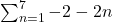Step-by-step explanation:

Arithmetic sequence:

In an arithmetic sequence, the difference of consecutive terms is always the same, called common difference.

The nth term of a sequence is given by: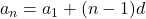In which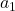is the first term and d is the common difference.

Sigma notation to represent the sum of the first seven terms

Sum going from the index starting at 1 and finishing at 7, that is: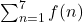Now we have to fund the function, which is given by an arithmetic sequence.

−4, −6, −8,

First term -4, common difference – 6 – (-4) = -6 + 4 = -2, so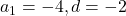Then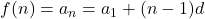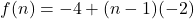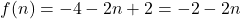Sigma notation:

Replacing f(n)